# How To Create A Random Number Generator In Google Sheets

The RAND function in Google Sheets generates a random number between 0 and 1 and can be used as a random number generator in Google Sheets.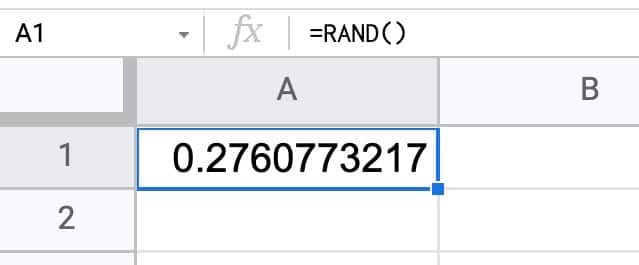The RAND function outputs a decimal number between 0 and 1, for example, 0.2760773217.

## RAND Function Notes

`=RAND()`

It takes no arguments.

The RAND function is a volatile function, which means it recalculates every time a change is made in your Sheet or every time you open your Google Sheet.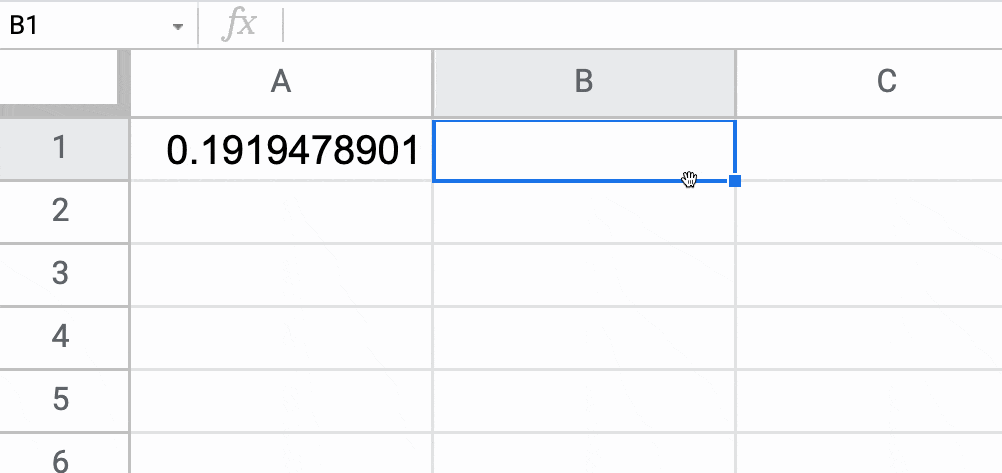If you have a lot of RAND functions in your Sheet (or a lot of downstream formulas that reference a RAND function), this can lead to slow Google Sheets because it will keep recalculating all those formulas with every change.

The RAND function can also be forced to recalculate every hour or every minute, which can be used as a trigger for other actions, as shown in this dynamic table of contents example.

To force recalculations, go to the menu: File > Settings > Calculation

Select the hour or minute options under the Recalculation dropdown menu: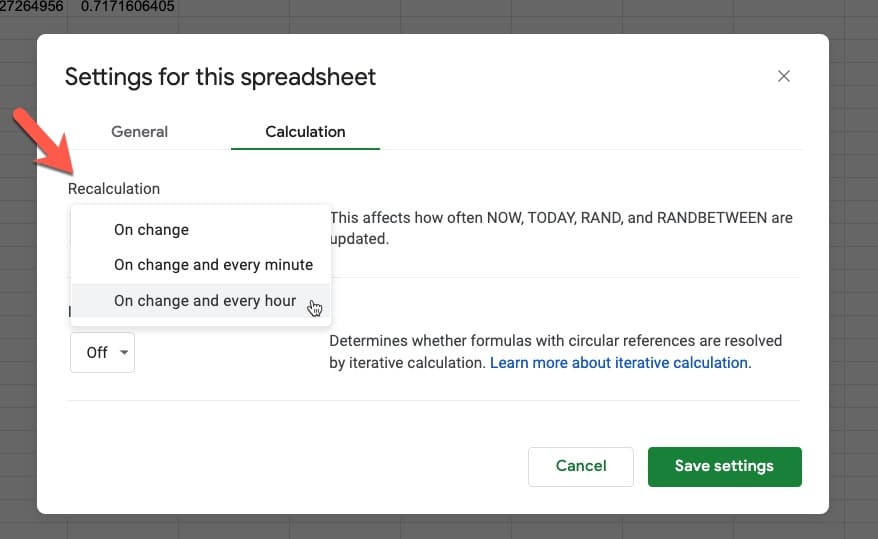## How To Generate A Random Number In Google Sheets Between Two Numbers

To generate a random number between two values, use this formula, where X and Y are the lower and upper boundaries:

`=RAND()*(Y-X)+X`

For example, to create a random number generator in Google Sheets between 1 and 50, use this formula:

`=RAND() * (50-1) + 1`

Note: To generate a random integer (i.e. a whole number) between two values, use the RANDBETWEEN function.

`=RANDBETWEEN(1, 50)`

will output an integer between 1 and 50, e.g. 26.

## Random Number Generator Referencing Other Cells

The lower and upper boundaries can be referenced in other cells, rather than written directly in the formula.

For example, with a lower bound in A1 and an upper bound in cell A2, this formula generates a random number between them:

`=RAND()*(A2-A1)+A1`

which looks like this in your Sheet: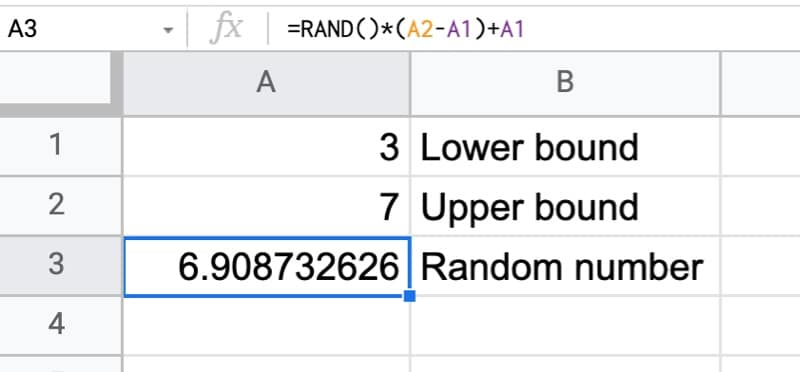## How To Create Random Arrays

Use the RANDARRAY function.

`=RANDARRAY(10,3)`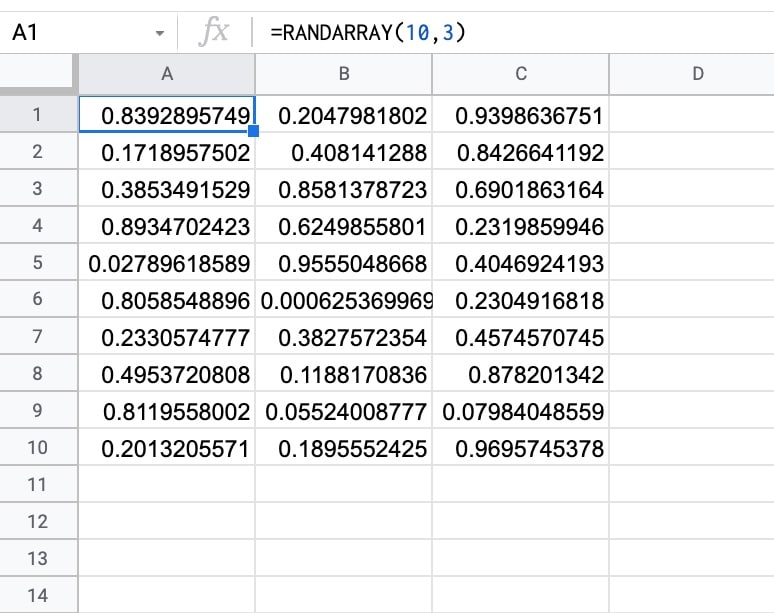## How To Create Random Number Between Two Values

Use the RANDBETWEEN function.

`=RANDBETWEEN(1,10)`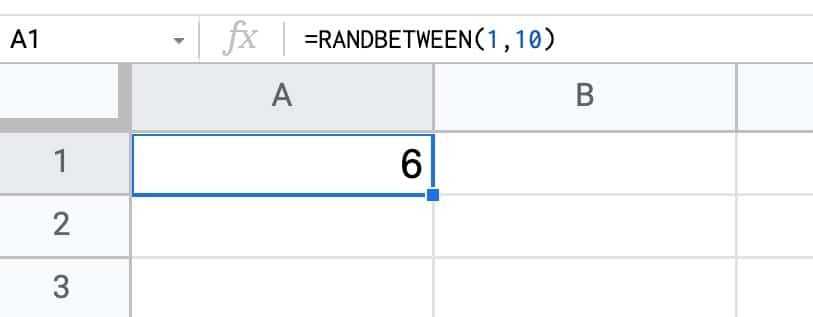## RAND Function Template

Feel free to make a copy: File > Make a copy

If you can’t access the template, it might be because of your organization’s Google Workspace settings. If you right-click the link and open it in an Incognito window you’ll be able to see it.

## 6 thoughts on “How To Create A Random Number Generator In Google Sheets”

1.Jake says:

Can you specify that certain cells do not duplicate a number? For example, if 5 cells are all =RANDBETWEEN(1,10), can you make each cell a unique number in that range and no duplicates?

This would allow me to make bingo cards with unique numbers each time and no duplicate numbers in any column on the same card.

1.Ben says:

Hey Jake,

Try this formula:

`=ARRAY_CONSTRAIN(SORT(HSTACK(SEQUENCE(10,1),RANDARRAY(10)),2,TRUE),5,1)`

You can change the 10’s in the formula to any other upper limit, e.g. 20 if you want the number to be between 1 and 20 say.

You can change the 5 to increase the number of rows (cannot be greater than the upper limit though).

Cheers,
Ben

2.Emily MB Laidlaw says:

I have a problem with using any of the rand functions. I have created games in google sheets that can be played cooperatively with students (I am a teacher and tutor). I can randomize lists and choose randome “cards” from a list using appscript, but the students can’t authorize the script. When I use the rand functions, the two students see a different random “card” because the rand formula is choosing a different random number on each device even though they are looking at the same document. Is there a was to use formulas that can make a shuffled list of numbers or words that does not change on each edit and each device?

1.Isabel Incorvati says:

Not a formula and I’m not sure if this would work, but you could copy all of the lists and ctrl+shift+v to paste just the values, creating a stable list that doesn’t change.

3.Kwame Qova says:

What algorithm supports the PRNG in google sheets?

4.Ian says:

Just curious, if I want to make a 6 digit combination that only has numbers between 1 to 6 (so excluding 7, 8, 9, 0), I’ll need to create 6 columns instead?